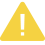# 百倍加速！Python量化策略的算法性能提升指南

### 性能问题

Python在2016年里可以说是风靡国内量化投资圈，目前整个生态链已经初具规模：

• 数据：tushare

• 回测：rqalpha

• 在线平台：UQER、RiceQuant、JoinQuant

（即使作为破旧自行车，我也深表怀疑这辆能不能骑好吧）

### 一段常用的代码

```# 这个测试目标在于仿造一个类似于实盘中，不断有新的数据推送过来，
# 然后需要计算移动平均线数值，这么一个比较常见的任务。

from __future__ import divisionimport timeimport random

# 生成测试用的数据
data = []
data_length = 100000    # 总数据量
ma_length = 500         # 移动均线的窗口
test_times = 10         # 测试次数for i in range(data_length):
data.append(random.randint(1, 100))```

```# 计算500期的移动均线，并将结果保存到一个列表里返回def ma_basic(data, ma_length):

# 用于保存均线输出结果的列表
ma = []

# 计算均线用的数据窗口
data_window = data[:ma_length]

# 测试用数据（去除了之前初始化用的部分）
test_data = data[ma_length:]

# 模拟实盘不断收到新数据推送的情景，遍历历史数据计算均线    for new_tick in test_data:
# 移除最老的数据点并增加最新的数据点
data_window.pop(0)
data_window.append(new_tick)

# 遍历求均线
sum_tick = 0
for tick in data_window:
sum_tick += tick
ma.append(sum_tick/ma_length)

# 返回数据    return ma

# 运行测试
start = time.time()for i in range(test_times):
result = ma_basic(data, ma_length)

time_per_test = (time.time()-start)/test_times
time_per_point = time_per_test/(data_length - ma_length)

print u'单次耗时：%s秒' %time_per_test
print u'单个数据点耗时：%s微秒' %(time_per_point*1000000)
print u'最后10个移动平均值：', result[-10:]```

ma_basic测试结果

• 单次耗时：1.15699999332秒

• 单个数据点耗时：11.6281406364微秒

### 试试NumPy？

```# 改用numpy（首先是一种常见的错误用法）import numpy as np

def ma_numpy_wrong(data, ma_length):
ma = []
data_window = data[:ma_length]
test_data = data[ma_length:]    for new_tick in test_data:
data_window.pop(0)
data_window.append(new_tick)

# 使用numpy求均线，注意这里本质上每次循环
# 都在创建一个新的numpy数组对象，开销很大
data_array = np.array(data_window)
ma.append(data_array.mean())    return ma```

ma_numpy_wrong测试结果

• 单次耗时：2.11879999638秒

• 单个数据点耗时：21.2944723254微秒

WTF?!用NumPy后居然反而速度降低了一半（耗时增加到了快2倍）！

data_array = np.array(data_window)

```# numpy的正确用法def ma_numpy_right(data, ma_length):
ma = []

# 用numpy数组来缓存计算窗口内的数据
data_window = np.array(data[:ma_length])

test_data = data[ma_length:]    for new_tick in test_data:
# 使用numpy数组的底层数据偏移来实现数据更新
data_window[0:ma_length-1] = data_window[1:ma_length]
data_window[-1] = new_tick
ma.append(data_window.mean())    return ma```

ma_numpy_right测试结果

• 单次耗时：0.614300012589秒

• 单个数据点耗时：6.17386947325微秒

### JIT神器：Numba

```# 使用numba加速，ma_numba函数和ma_basic完全一样import numba

@numba.jit
def ma_numba(data, ma_length):
ma = []
data_window = data[:ma_length]
test_data = data[ma_length:]for new_tick in test_data:
data_window.pop(0)
data_window.append(new_tick)
sum_tick = 0
for tick in data_window:
sum_tick += tick
ma.append(sum_tick/ma_length)return ma```

ma_numba测试结果

• 单次耗时：0.043700003624秒

• 单个数据点耗时：0.439196016321微秒

OMG！就加了一行@numba.jit，性能竟然提高了26倍！这估计是按照代码修改行数算，性价比最高的优化方案了。

### 改写算法

```# 将均线计算改写为高速算法def ma_online(data, ma_length):
ma = []
data_window = data[:ma_length]
test_data = data[ma_length:]

# 缓存的窗口内数据求和结果
sum_buffer = 0

for new_tick in test_data:
old_tick = data_window.pop(0)
data_window.append(new_tick)

# 如果缓存结果为空，则先通过遍历求第一次结果        if not sum_buffer:
sum_tick = 0
for tick in data_window:
sum_tick += tick
ma.append(sum_tick/ma_length)

# 将求和结果缓存下来
sum_buffer = sum_tick        else:
# 这里的算法将计算复杂度从O(n)降低到了O(1)
sum_buffer = sum_buffer - old_tick + new_tick
ma.append(sum_buffer/ma_length)    return ma```

ma_online测试结果

• 单次耗时：0.0348000049591秒

• 单个数据点耗时：0.349748793559微秒

(索罗斯：其实我是个哲学家。)

```# 高速算法和numba结合，ma_online_numba函数和ma_online完全一样
@numba.jit
def ma_online_numba(data, ma_length):
ma = []
data_window = data[:ma_length]
test_data = data[ma_length:]

sum_buffer = 0

for new_tick in test_data:
old_tick = data_window.pop(0)
data_window.append(new_tick)        if not sum_buffer:
sum_tick = 0
for tick in data_window:
sum_tick += tick
ma.append(sum_tick/ma_length)
sum_buffer = sum_tick        else:
sum_buffer = sum_buffer - old_tick + new_tick
ma.append(sum_buffer/ma_length)    return ma```

ma_online_numba测试结果

• 单次耗时：0.0290000200272秒

• 单个数据点耗时：0.29145748771微秒

### 终极武器：Cython

```# 基础的cython加速def ma_cython(data, ma_length):
ma = []
data_window = data[:ma_length]
test_data = data[ma_length:]    for new_tick in test_data:
data_window.pop(0)
data_window.append(new_tick)

sum_tick = 0
for tick in data_window:
sum_tick += tick
ma.append(sum_tick/ma_length)    return ma```

ma_cython测试结果

• 单次耗时：0.600800013542秒

• 单个数据点耗时：6.03819109088微秒

ma_cython和ma_basic的代码完全相同，简单使用Cython编译后性能提高了大约1倍，不过这和之前我们已经达成的优化效果比可以说是毫无吸引力。

Cython官方的Quick Start里，第一步是教会用户如何去编译程序，第二步就是如何使用静态声明来大幅提高性能，所以我们的下一步就是：静态声明+高速算法。

```# cython和高速算法def ma_cython_online(data, ma_length):
# 静态声明变量
cdef int sum_buffer, sum_tick, old_tick, new_tick

ma = []
data_window = data[:ma_length]
test_data = data[ma_length:]
sum_buffer = 0

for new_tick in test_data:
old_tick = data_window.pop(0)
data_window.append(new_tick)        if not sum_buffer:
sum_tick = 0
for tick in data_window:
sum_tick += tick
ma.append(sum_tick/ma_length)

sum_buffer = sum_tick        else:
sum_buffer = sum_buffer - old_tick + new_tick
ma.append(sum_buffer/ma_length)    return ma```

ma_cython_online测试结果

• 单次耗时：0.00980000495911秒

• 单个数据点耗时：0.0984925121518微秒

117倍！！！比ma_online_numba的速度还提高了接近3倍，98纳秒的计算速度已经足以满足大部分毫秒级别高频策略的延时需求。

cdef int sum_buffer, sum_tick, old_tick, new_tick

• 把要使用Cython编译的函数放到.pyx文件中，比如test.pyx

• 创建setup.py用于设置相关编译选项

• 打开cmd或者terminal进入到test.pyx和setup.py所在的文件夹

• 运行python setup.py build_ext --inplace，执行编译

• 若编译成功则在当前文件夹下会出现test.pyd

• 打开python像使用其他模块一样载入（import）test.pyd使用

### 写在最后

• 现实工作中遇到需要优化Python程序性能时，首先要做的就是去寻找程序里延时较大的热点代码，找到了问题所在，解决方案才有意义；

• 所有的优化工作都应该基于测试来一步步推进，同样的优化方法对于不同类型的代码效果可能是截然相反的，同时错误的优化方法还不如不要优化（比如ma_numpy_wrong）；

• 只需增加一句代码（@numba.jit）就能实现加速的Numba无疑是性价比最高的优化方案，值得优先尝试，不过需要注意numba的JIT技术局限性比较大（主要针对数值计算相关的逻辑）；

• 学习如何降低算法复杂度和编写更高效的算法，可以在潜移默化中提高自己的编程水平，在长期而言是对Quant或者程序员最有价值的优化方法；

• 如果其他优化方法都无法达到令你满意的性能水平，试试Cython（记得一定要加静态声明）;

• 一个好的程序架构设计非常重要，把功能不同的计算逻辑分解到不同的函数里，适当降低每个函数的代码行数，会有助于后期的性能优化工作。

【版权声明】本文为华为云社区用户转载文章，如果您发现本社区中有涉嫌抄袭的内容，欢迎发送邮件至：huaweicloud.bbs@huawei.com进行举报，并提供相关证据，一经查实，本社区将立刻删除涉嫌侵权内容。

### 评论 (0)

###### 温馨提示